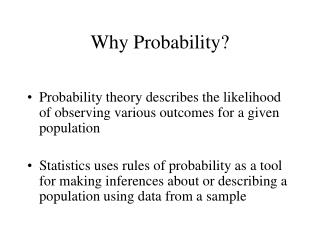# Why Probability? - PowerPoint PPT PresentationDownload PresentationWhy Probability?

Why Probability?
Download Presentation## Why Probability?

- - - - - - - - - - - - - - - - - - - - - - - - - - - E N D - - - - - - - - - - - - - - - - - - - - - - - - - - -
##### Presentation Transcript

1. Why Probability? • Probability theory describes the likelihood of observing various outcomes for a given population • Statistics uses rules of probability as a tool for making inferences about or describing a population using data from a sample

2. Some Concepts • Experiment: the process by which an observation is obtained • e.g. the roll of a dice • Event: the outcome of an experiment. • e.g. observe a 1; observe an odd number (1,3,5) • Simple Event: an event that cannot be decomposed • Sample Space (S): set of all events

3. Definition of Probability • The probability of an event A is a measure of our belief that the experiment will result in event A. • If we repeat an experiment N times, and event A occurs n times, then P(A) = n / N • Computing probabilities in this way is infeasible in practice, but implications are useful

4. Probability Rules For an event A: • P(A) is between 0 and 1, inclusive • If A contains t simple events, then P(A) = P(E1) + P(E2) + … + P(Et) For a sample space S with s simple events: P(S) = P(E1) + P(E2) + … + P(Es) = 1

5. Event Composition • The Intersection of events A and B is the event that both A and B occur • denoted AB or A∩B • The Union of events A and B is the event that A or B or both occur • denoted AUB

6. Event Composition • A and B are mutually exclusive if there are no simple events in A∩B. If A,B are mutually exclusive then: (1) P(A∩B) = 0 (2) P(AUB) = P(A) + P(B) • The complement of an event A consists of all simple events that are not in A • denoted

7. Conditional Probability • In some cases events are related, so that if we know event A has occurred then we learn more about an event B • Example: Roll a die A: observe an even number (2,4,6) B: observe a number less than 4 (1,2,3) if we know nothing else then P(B) = 3/6 = 1/2 But if we know A has occurred then P(B | A) = 1/3

8. Conditional Probability • More generally, we can express the conditional probability of B given that A has occurred as: • We can rewrite this formula to get the Multiplicative Rule of Probability:

9. Independence • Events are not always be related. Events A and B are independent if and only if: • If A and B are independent, then from Multiplicative Rule of Probability:

10. Rules of Probability Given 2 events A and B: • Additive Probability: • If A and B are mutually exclusive then • P(AB)=0 • P(A+B) = P ( A )+ P ( B ) • Total Probability: for mutually exclusive B1, B2, …

11. Bayes Rule • Take into account prior information when computing probabilities • Let S1, S2, S3,…Sk represent k mutually exclusive, only possible states of nature with prior probabilities P(S1), P(S2),…P(Sk). If an event A occurs, the posterior probability of Si given A is the conditional probability

12. Random Variables • X is a random variable if value that it assumes depends on the random outcome of an experiment • A random variable may be • Discrete: countable number of values • Continuous: infinite number of values

13. Discrete Probability Distribution • The probability distribution for a discrete random variable is a formula, table or graph that provides p(x), the probability associated with observing x • Rules for probability distribution: • 0 <= p(x) <= 1 • ∑ p(x) = 1

14. Expected Value • Expected value (or population mean) of a random variable x with the probability distribution p(x) is Intuition:expected value is weighted average of x

15. Variance of a Random Variable • The variance of random variable x with probability distribution p(x) and expected value E(x)= is given as • The Standard Deviation of random variable x is equal to the square root of its variance.